# What is Cluster Analysis Cluster a collection of

• Slides: 51What is Cluster Analysis? • Cluster: a collection of data objects – Similar to one another within the same cluster – Dissimilar to the objects in other clusters • Cluster analysis – Finding similarities between data according to the characteristics found in the data and grouping similar data objects into clusters • Unsupervised learning: no predefined classes • Typical applications – As a stand-alone tool to get insight into data distribution – As a preprocessing step for other algorithms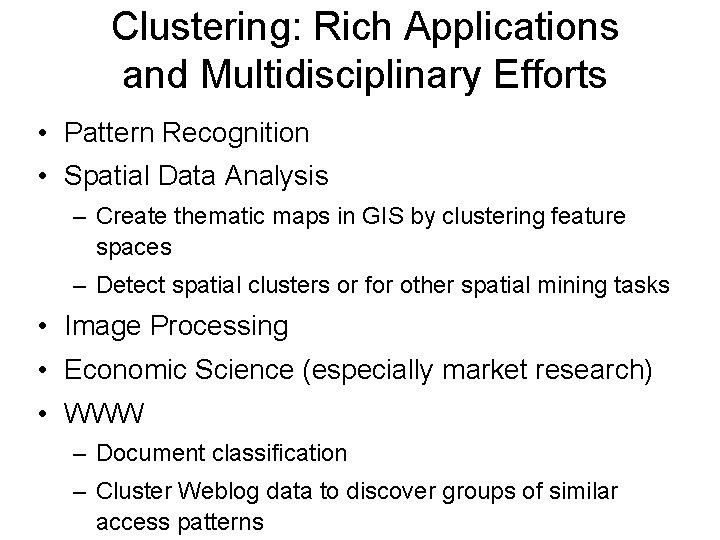Clustering: Rich Applications and Multidisciplinary Efforts • Pattern Recognition • Spatial Data Analysis – Create thematic maps in GIS by clustering feature spaces – Detect spatial clusters or for other spatial mining tasks • Image Processing • Economic Science (especially market research) • WWW – Document classification – Cluster Weblog data to discover groups of similar access patterns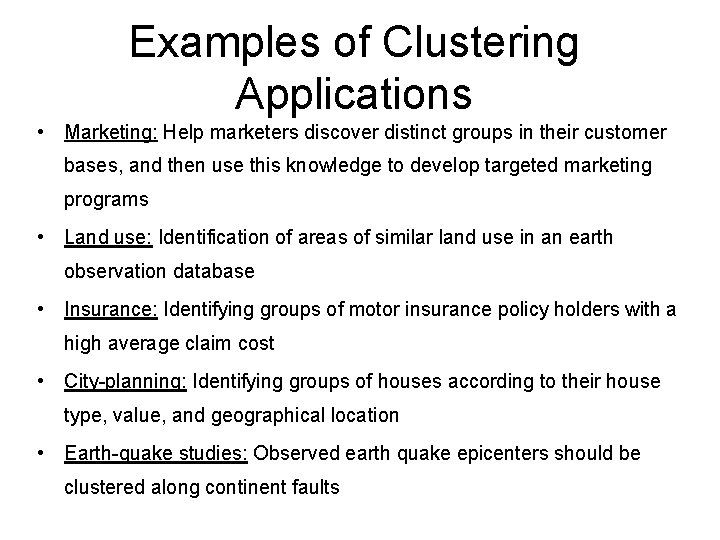Examples of Clustering Applications • Marketing: Help marketers discover distinct groups in their customer bases, and then use this knowledge to develop targeted marketing programs • Land use: Identification of areas of similar land use in an earth observation database • Insurance: Identifying groups of motor insurance policy holders with a high average claim cost • City-planning: Identifying groups of houses according to their house type, value, and geographical location • Earth-quake studies: Observed earth quake epicenters should be clustered along continent faults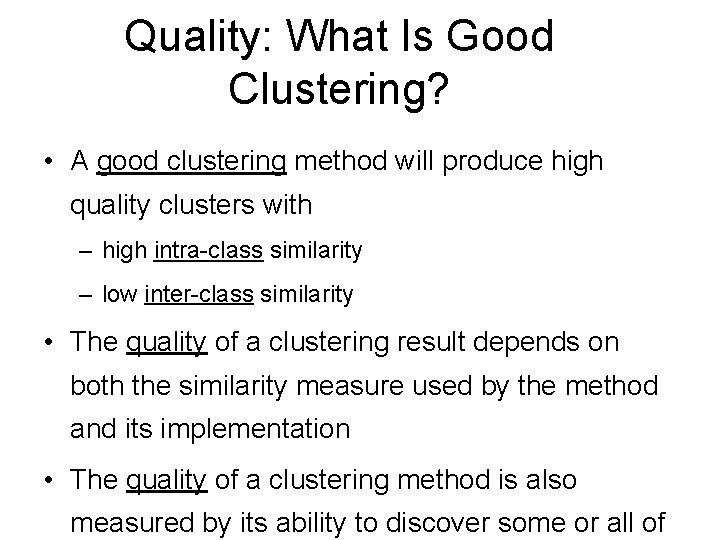Quality: What Is Good Clustering? • A good clustering method will produce high quality clusters with – high intra-class similarity – low inter-class similarity • The quality of a clustering result depends on both the similarity measure used by the method and its implementation • The quality of a clustering method is also measured by its ability to discover some or all of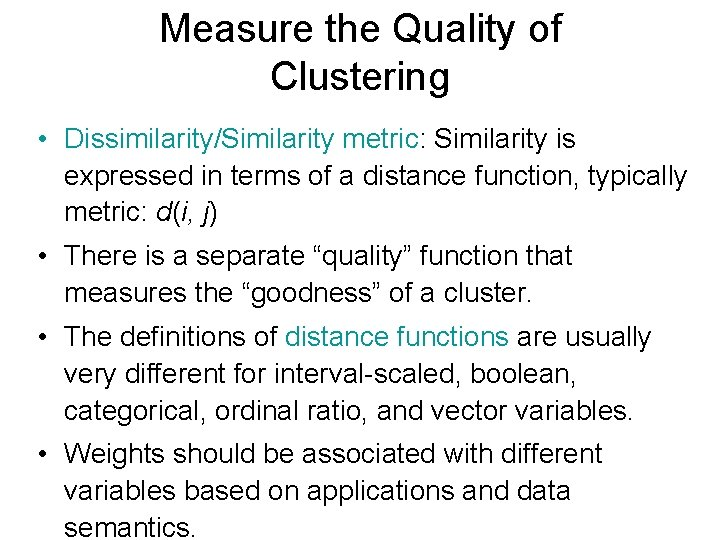Measure the Quality of Clustering • Dissimilarity/Similarity metric: Similarity is expressed in terms of a distance function, typically metric: d(i, j) • There is a separate “quality” function that measures the “goodness” of a cluster. • The definitions of distance functions are usually very different for interval-scaled, boolean, categorical, ordinal ratio, and vector variables. • Weights should be associated with different variables based on applications and data semantics.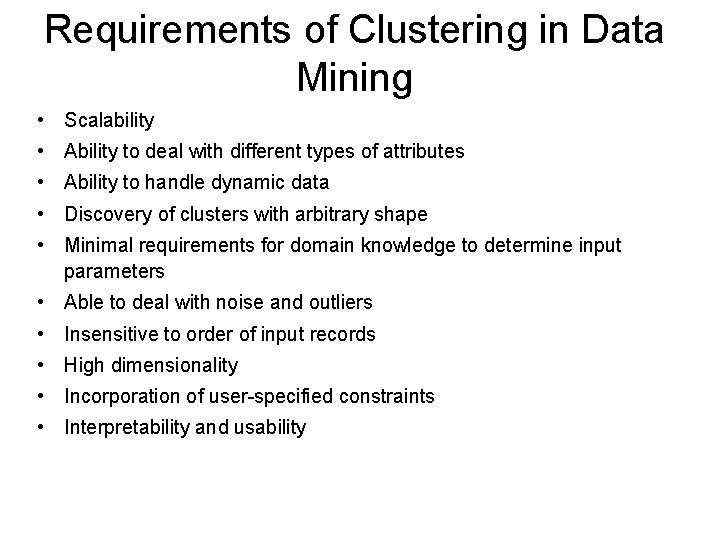Requirements of Clustering in Data Mining • Scalability • Ability to deal with different types of attributes • Ability to handle dynamic data • Discovery of clusters with arbitrary shape • Minimal requirements for domain knowledge to determine input parameters • Able to deal with noise and outliers • Insensitive to order of input records • High dimensionality • Incorporation of user-specified constraints • Interpretability and usability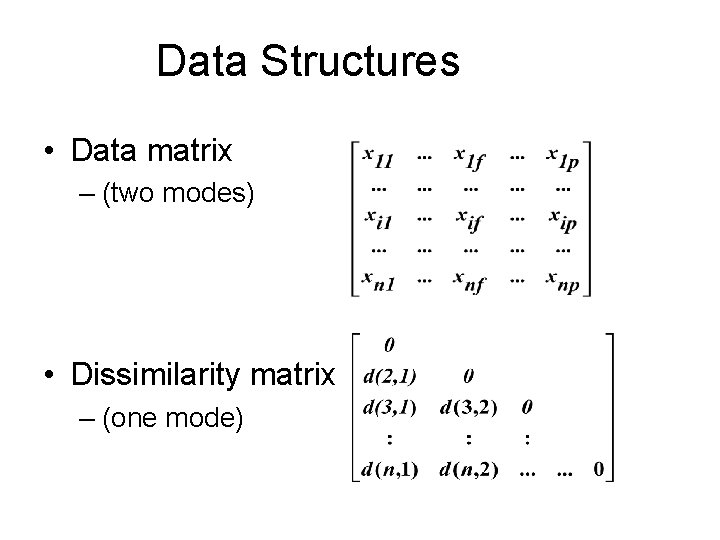Data Structures • Data matrix – (two modes) • Dissimilarity matrix – (one mode)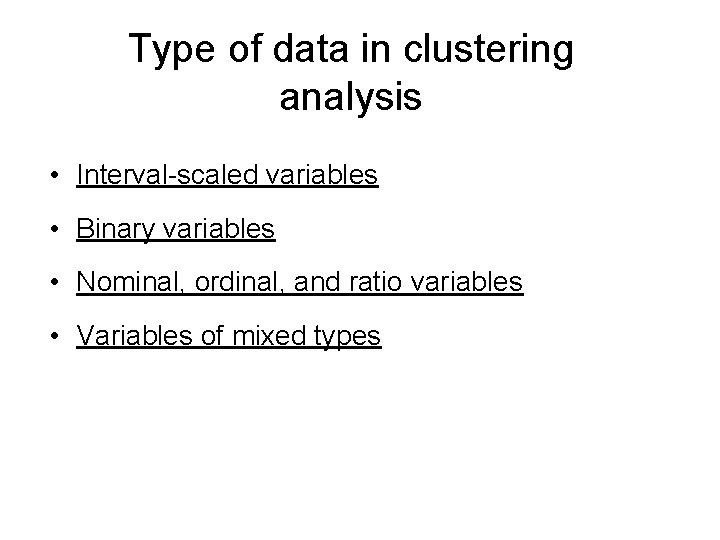Type of data in clustering analysis • Interval-scaled variables • Binary variables • Nominal, ordinal, and ratio variables • Variables of mixed types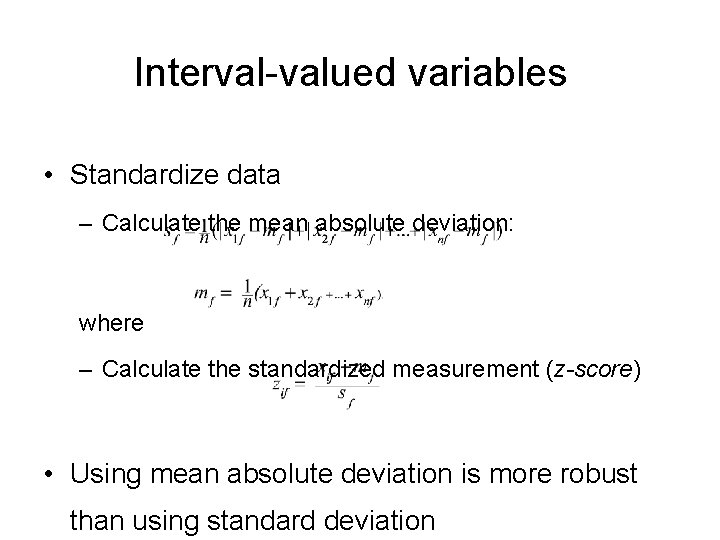Interval-valued variables • Standardize data – Calculate the mean absolute deviation: where – Calculate the standardized measurement (z-score) • Using mean absolute deviation is more robust than using standard deviationSimilarity and Dissimilarity Between Objects • Distances are normally used to measure the similarity or dissimilarity between two data objects • Some popular ones include: Minkowski distance: where i = (xi 1, xi 2, …, xip) and j = (xj 1, xj 2, …, xjp) are two p-dimensional data objects, and q is a positive integerSimilarity and Dissimilarity Between Objects (Cont. ) • If q = 2, d is Euclidean distance: – Properties • d(i, j) 0 • d(i, i) = 0 • d(i, j) = d(j, i) • d(i, j) d(i, k) + d(k, j) • Also, one can use weighted distance, parametric Pearson product moment correlation, or other disimilarity measures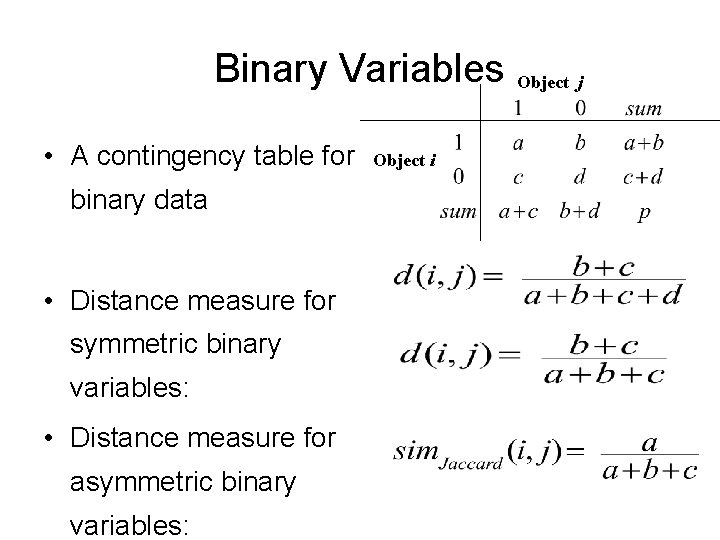Binary Variables Object j • A contingency table for binary data • Distance measure for symmetric binary variables: • Distance measure for asymmetric binary variables: Object i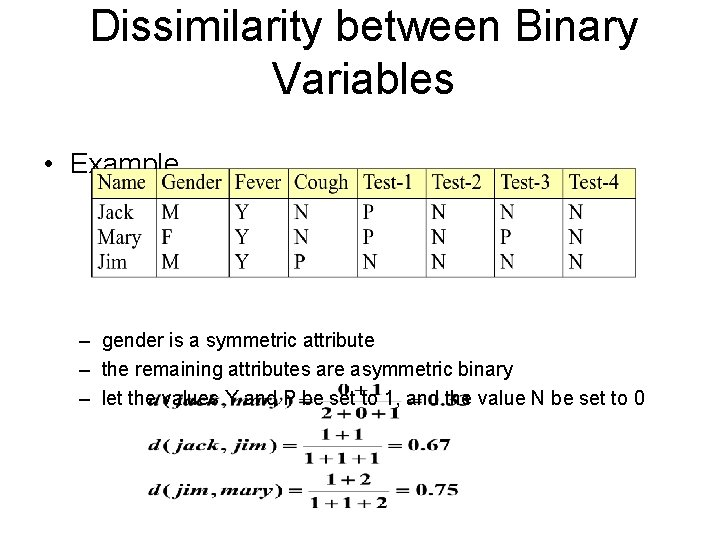Dissimilarity between Binary Variables • Example – gender is a symmetric attribute – the remaining attributes are asymmetric binary – let the values Y and P be set to 1, and the value N be set to 0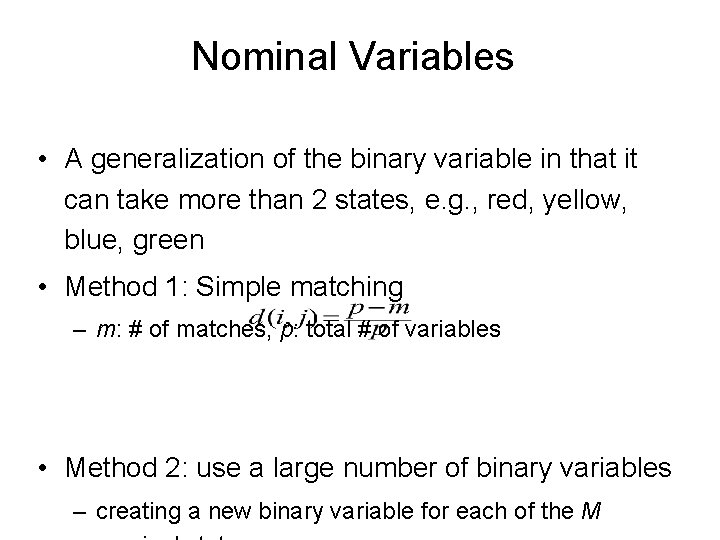Nominal Variables • A generalization of the binary variable in that it can take more than 2 states, e. g. , red, yellow, blue, green • Method 1: Simple matching – m: # of matches, p: total # of variables • Method 2: use a large number of binary variables – creating a new binary variable for each of the MOrdinal Variables • An ordinal variable can be discrete or continuous • Order is important, e. g. , rank • Can be treated like interval-scaled – replace xif by their rank – map the range of each variable onto [0, 1] by replacing i -th object in the f-th variable by – compute the dissimilarity using methods for intervalscaled variablesRatio-Scaled Variables • Ratio-scaled variable: a positive measurement on a nonlinear scale, approximately at exponential scale, such as Ae. Bt or Ae-Bt • Methods: – treat them like interval-scaled variables—not a good choice! (why? —the scale can be distorted) – apply logarithmic transformation yif = log(xif) – treat them as continuous ordinal data treat their rank as interval-scaledVariables of Mixed Types • A database may contain all the six types of variables – symmetric binary, asymmetric binary, nominal, ordinal, interval and ratio • One may use a weighted formula to combine their effects – f is binary or nominal: dij(f) = 0 if xif = xjf , or dij(f) = 1 otherwise – f is interval-based: use the normalized distance – f is ordinal or ratio-scaled • compute ranks rif and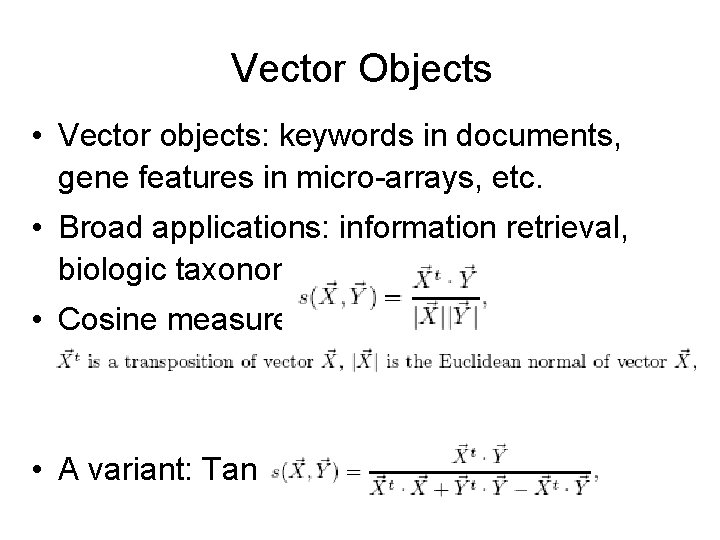Vector Objects • Vector objects: keywords in documents, gene features in micro-arrays, etc. • Broad applications: information retrieval, biologic taxonomy, etc. • Cosine measure • A variant: Tanimoto coefficientMajor Clustering Approaches (I) • Partitioning approach: – Construct various partitions and then evaluate them by some criterion, e. g. , minimizing the sum of square errors – Typical methods: k-means, k-medoids, CLARANS • Hierarchical approach: – Create a hierarchical decomposition of the set of data (or objects) using some criterion – Typical methods: Diana, Agnes, BIRCH, ROCK, CAMELEON • Density-based approach: – Based on connectivity and density functions – Typical methods: DBSACN, OPTICS, Den. Clue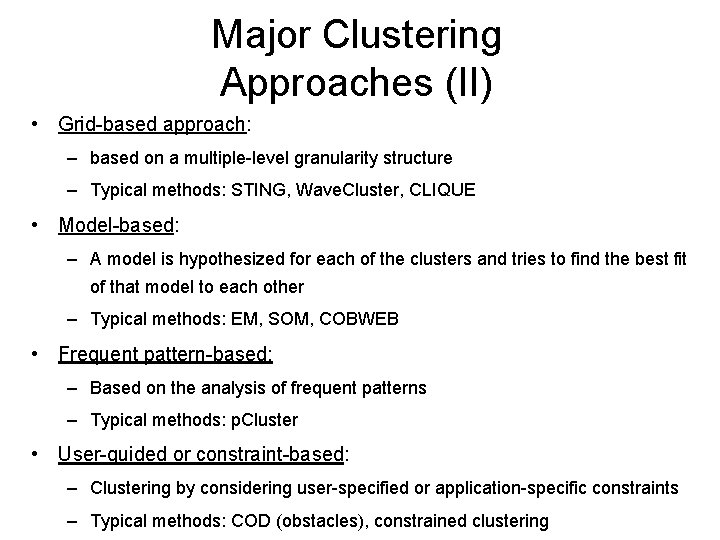Major Clustering Approaches (II) • Grid-based approach: – based on a multiple-level granularity structure – Typical methods: STING, Wave. Cluster, CLIQUE • Model-based: – A model is hypothesized for each of the clusters and tries to find the best fit of that model to each other – Typical methods: EM, SOM, COBWEB • Frequent pattern-based: – Based on the analysis of frequent patterns – Typical methods: p. Cluster • User-guided or constraint-based: – Clustering by considering user-specified or application-specific constraints – Typical methods: COD (obstacles), constrained clusteringTypical Alternatives to Calculate the Distance between Clusters • Single link: smallest distance between an element in one cluster and an element in the other, i. e. , dis(Ki, Kj) = min(tip, tjq) • Complete link: largest distance between an element in one cluster and an element in the other, i. e. , dis(Ki, Kj) = max(tip, tjq) • Average: avg distance between an element in one cluster and an element in the other, i. e. , dis(Ki, Kj) = avg(tip, tjq) • Centroid: distance between the centroids of two clusters,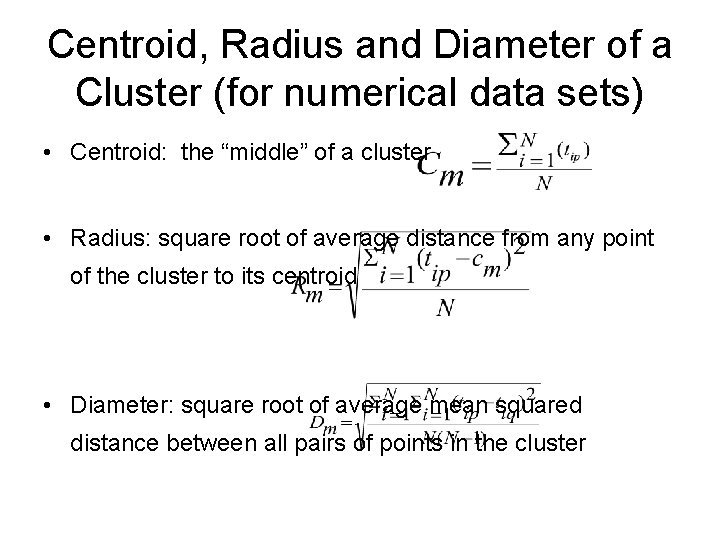Centroid, Radius and Diameter of a Cluster (for numerical data sets) • Centroid: the “middle” of a cluster • Radius: square root of average distance from any point of the cluster to its centroid • Diameter: square root of average mean squared distance between all pairs of points in the cluster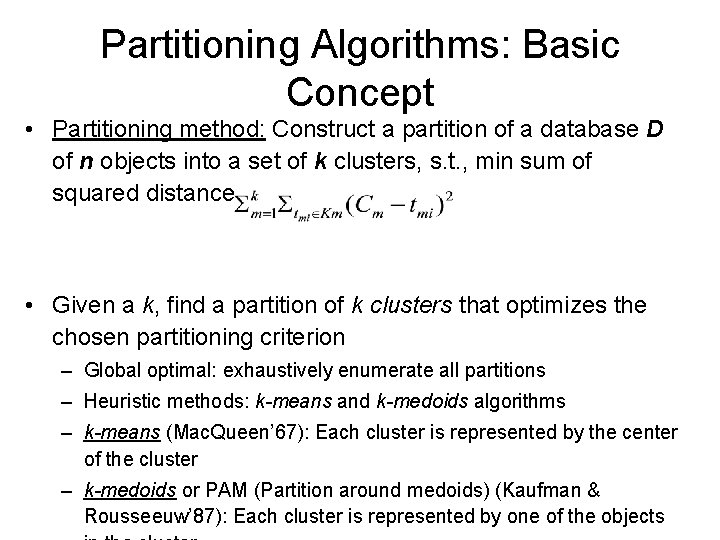Partitioning Algorithms: Basic Concept • Partitioning method: Construct a partition of a database D of n objects into a set of k clusters, s. t. , min sum of squared distance • Given a k, find a partition of k clusters that optimizes the chosen partitioning criterion – Global optimal: exhaustively enumerate all partitions – Heuristic methods: k-means and k-medoids algorithms – k-means (Mac. Queen’ 67): Each cluster is represented by the center of the cluster – k-medoids or PAM (Partition around medoids) (Kaufman & Rousseeuw’ 87): Each cluster is represented by one of the objectsThe K-Means Clustering Method • Given k, the k-means algorithm is implemented in four steps: – Partition objects into k nonempty subsets – Compute seed points as the centroids of the clusters of the current partition (the centroid is the center, i. e. , mean point, of the cluster) – Assign each object to the cluster with the nearest seed point – Go back to Step 2, stop when no more new assignment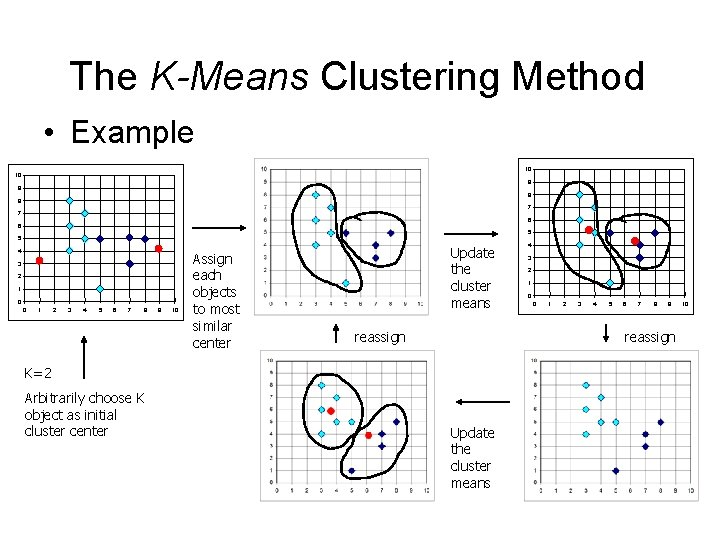The K-Means Clustering Method • Example 10 10 9 9 8 8 7 7 6 6 5 5 4 3 2 1 0 0 1 2 3 4 5 6 7 8 9 10 Assign each objects to most similar center Update the cluster means reassign 3 2 1 0 0 1 2 3 4 5 6 7 8 9 reassign K=2 Arbitrarily choose K object as initial cluster center 4 Update the cluster means 10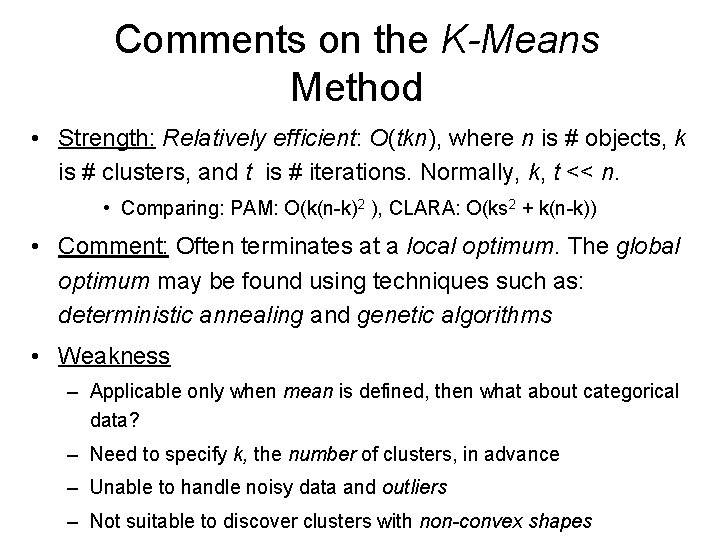Comments on the K-Means Method • Strength: Relatively efficient: O(tkn), where n is # objects, k is # clusters, and t is # iterations. Normally, k, t << n. • Comparing: PAM: O(k(n-k)2 ), CLARA: O(ks 2 + k(n-k)) • Comment: Often terminates at a local optimum. The global optimum may be found using techniques such as: deterministic annealing and genetic algorithms • Weakness – Applicable only when mean is defined, then what about categorical data? – Need to specify k, the number of clusters, in advance – Unable to handle noisy data and outliers – Not suitable to discover clusters with non-convex shapesVariations of the K-Means Method • A few variants of the k-means which differ in – Selection of the initial k means – Dissimilarity calculations – Strategies to calculate cluster means • Handling categorical data: k-modes (Huang’ 98) – Replacing means of clusters with modes – Using new dissimilarity measures to deal with categorical objects – Using a frequency-based method to update modes of clusters – A mixture of categorical and numerical data: k-prototype methodWhat Is the Problem of the K-Means Method? • The k-means algorithm is sensitive to outliers ! – Since an object with an extremely large value may substantially distort the distribution of the data. • K-Medoids: Instead of taking the mean value of the object in a cluster as a reference point, medoids can be used, which is the most centrally located object in a cluster. 10 10 9 9 8 8 7 7 6 6 5 5 4 4 3 3 2 2 1 1 0 0 0 1 2 3 4 5 6 7 8 9 10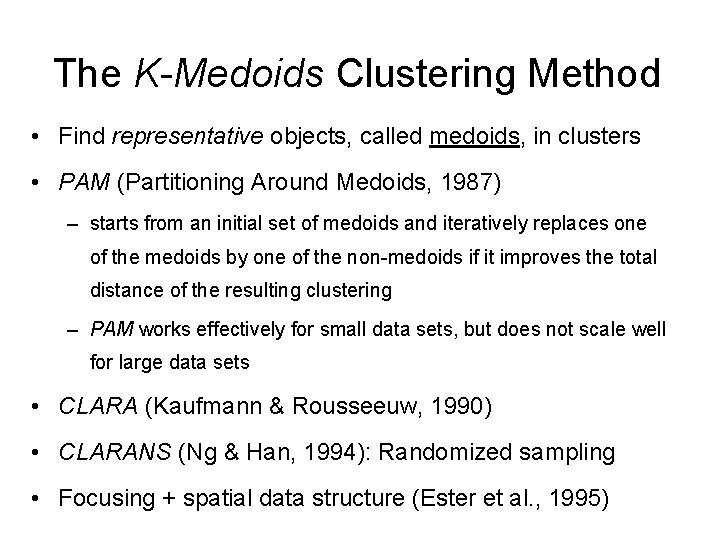The K-Medoids Clustering Method • Find representative objects, called medoids, in clusters • PAM (Partitioning Around Medoids, 1987) – starts from an initial set of medoids and iteratively replaces one of the medoids by one of the non-medoids if it improves the total distance of the resulting clustering – PAM works effectively for small data sets, but does not scale well for large data sets • CLARA (Kaufmann & Rousseeuw, 1990) • CLARANS (Ng & Han, 1994): Randomized sampling • Focusing + spatial data structure (Ester et al. , 1995)A Typical K-Medoids Algorithm (PAM) Total Cost = 20 10 9 8 Arbitrary choose k object as initial medoids 7 6 5 4 3 2 Assign each remainin g object to nearest medoids 1 0 0 1 2 3 4 5 6 7 8 9 10 K=2 Randomly select a nonmedoid object, Oramdom Total Cost = 26 Do loop Until no change 10 10 9 Swapping O and Oramdom If quality is improved. Compute total cost of swapping 8 7 6 9 8 7 6 5 5 4 4 3 3 2 2 1 1 0 0 0 1 2 3 4 5 6 7 8 9 10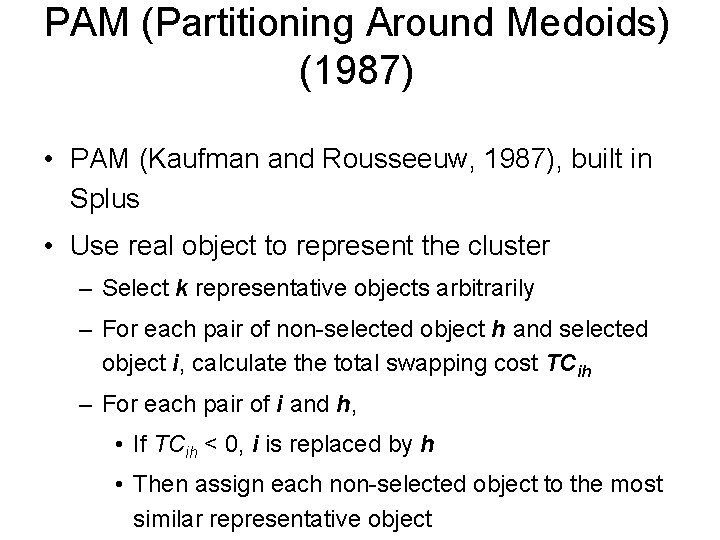PAM (Partitioning Around Medoids) (1987) • PAM (Kaufman and Rousseeuw, 1987), built in Splus • Use real object to represent the cluster – Select k representative objects arbitrarily – For each pair of non-selected object h and selected object i, calculate the total swapping cost TCih – For each pair of i and h, • If TCih < 0, i is replaced by h • Then assign each non-selected object to the most similar representative object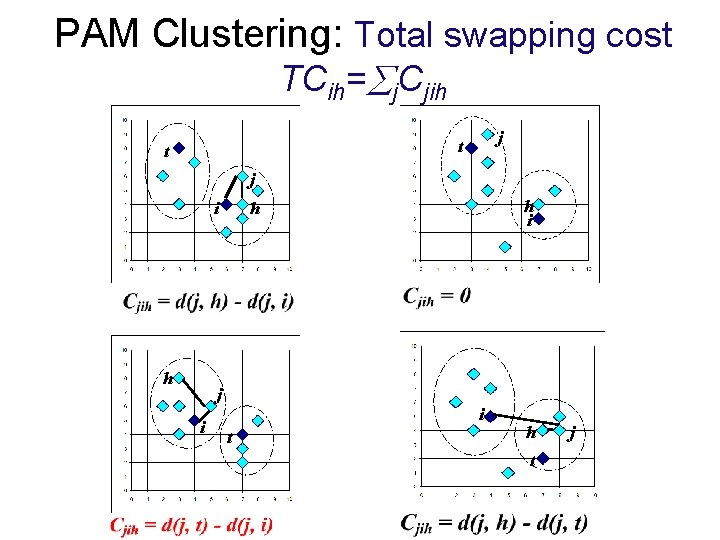PAM Clustering: Total swapping cost TCih= j. Cjih j t t j i h i h i t h t j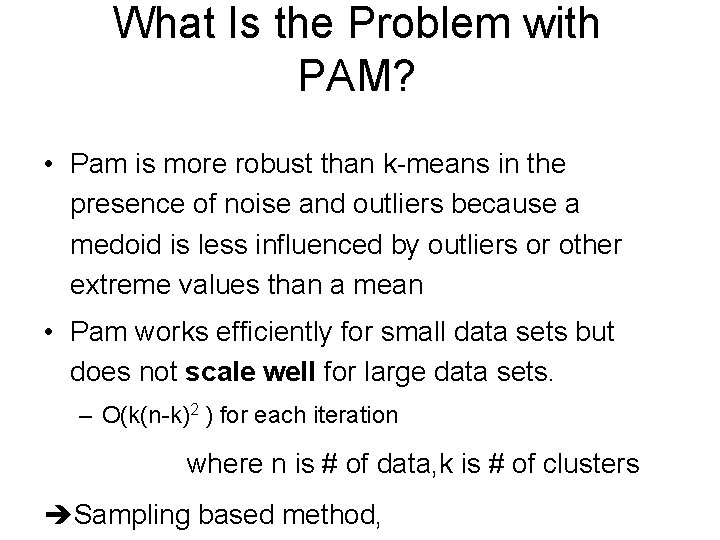What Is the Problem with PAM? • Pam is more robust than k-means in the presence of noise and outliers because a medoid is less influenced by outliers or other extreme values than a mean • Pam works efficiently for small data sets but does not scale well for large data sets. – O(k(n-k)2 ) for each iteration where n is # of data, k is # of clusters èSampling based method,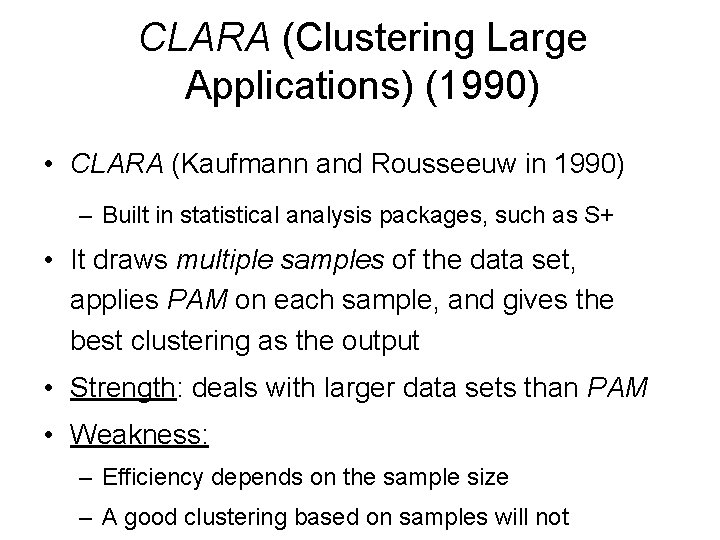CLARA (Clustering Large Applications) (1990) • CLARA (Kaufmann and Rousseeuw in 1990) – Built in statistical analysis packages, such as S+ • It draws multiple samples of the data set, applies PAM on each sample, and gives the best clustering as the output • Strength: deals with larger data sets than PAM • Weakness: – Efficiency depends on the sample size – A good clustering based on samples will not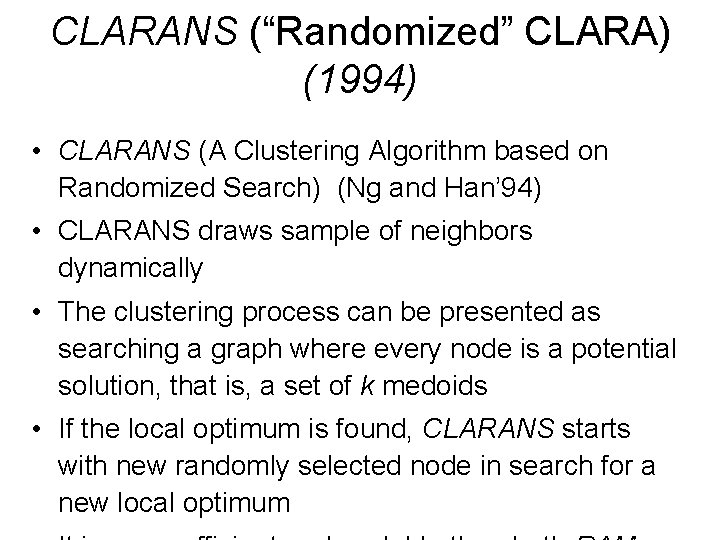CLARANS (“Randomized” CLARA) (1994) • CLARANS (A Clustering Algorithm based on Randomized Search) (Ng and Han’ 94) • CLARANS draws sample of neighbors dynamically • The clustering process can be presented as searching a graph where every node is a potential solution, that is, a set of k medoids • If the local optimum is found, CLARANS starts with new randomly selected node in search for a new local optimumHierarchical Clustering • Use distance matrix as clustering criteria. This method does not require the number of clusters k as an input, but needs a termination condition Step 0 a b Step 1 Step 2 Step 3 Step 4 ab abcde c cde d de e Step 4 agglomerative (AGNES) Step 3 Step 2 Step 1 Step 0 divisive (DIANA)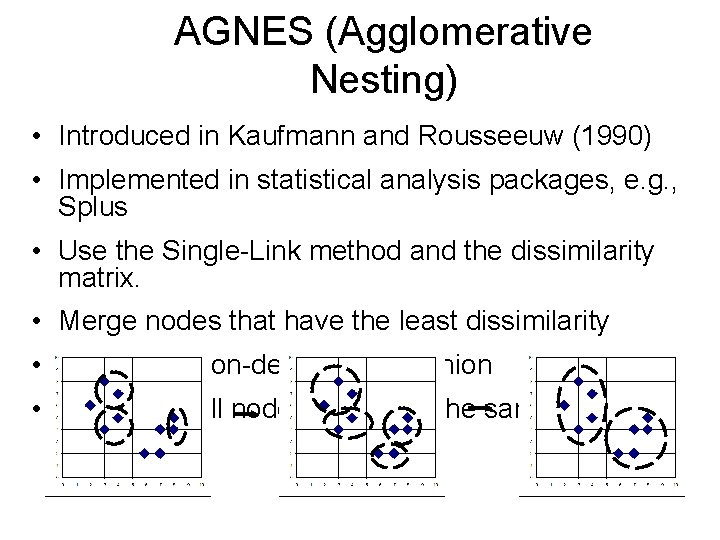AGNES (Agglomerative Nesting) • Introduced in Kaufmann and Rousseeuw (1990) • Implemented in statistical analysis packages, e. g. , Splus • Use the Single-Link method and the dissimilarity matrix. • Merge nodes that have the least dissimilarity • Go on in a non-descending fashion • Eventually all nodes belong to the same clusterDendrogram: Shows How the Clusters are Merged Decompose data objects into a several levels of nested partitioning (tree of clusters), called a dendrogram. A clustering of the data objects is obtained by cutting the dendrogram at the desired level, then each connected component forms a cluster.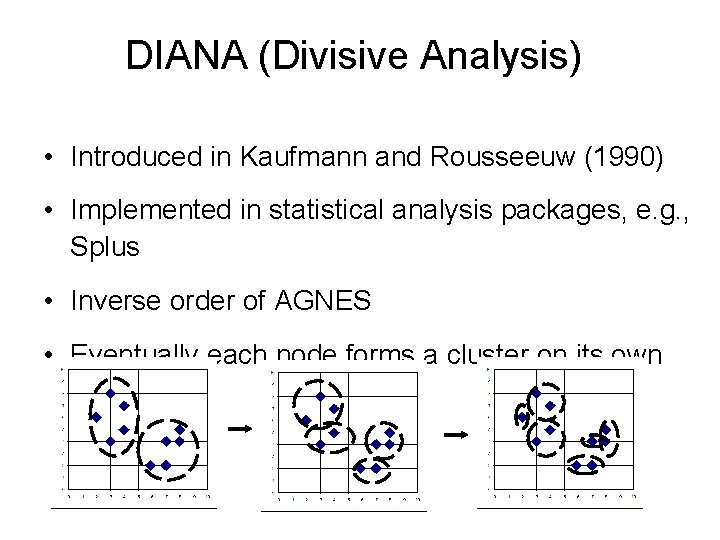DIANA (Divisive Analysis) • Introduced in Kaufmann and Rousseeuw (1990) • Implemented in statistical analysis packages, e. g. , Splus • Inverse order of AGNES • Eventually each node forms a cluster on its own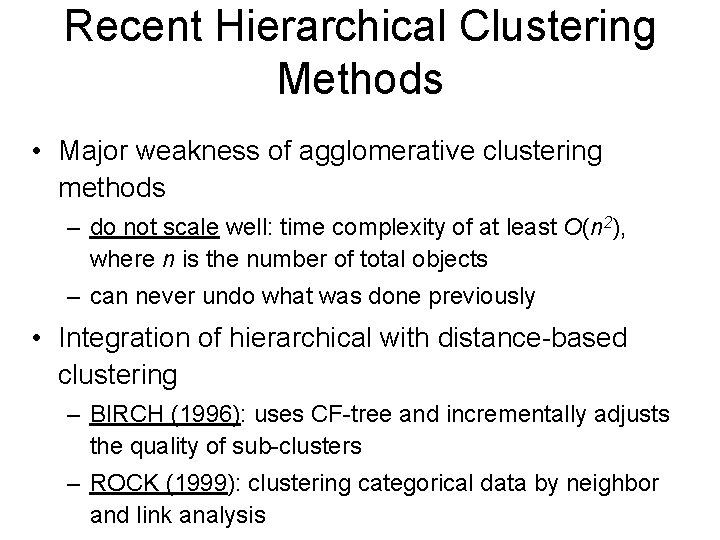Recent Hierarchical Clustering Methods • Major weakness of agglomerative clustering methods – do not scale well: time complexity of at least O(n 2), where n is the number of total objects – can never undo what was done previously • Integration of hierarchical with distance-based clustering – BIRCH (1996): uses CF-tree and incrementally adjusts the quality of sub-clusters – ROCK (1999): clustering categorical data by neighbor and link analysisClustering High-Dimensional Data • Clustering high-dimensional data – Many applications: text documents, DNA micro-array data – Major challenges: • Many irrelevant dimensions may mask clusters • Distance measure becomes meaningless—due to equi-distance • Clusters may exist only in some subspaces • Methods – Feature transformation: only effective if most dimensions are relevant • PCA & SVD useful only when features are highly correlated/redundant – Feature selection: wrapper or filter approaches • useful to find a subspace where the data have nice clusters – Subspace-clustering: find clusters in all the possible subspaces • CLIQUE, Pro. Clus, and frequent pattern-based clustering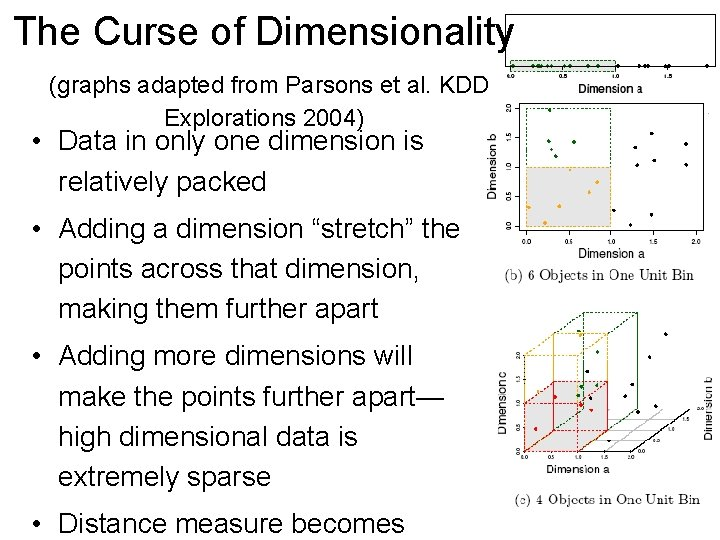The Curse of Dimensionality (graphs adapted from Parsons et al. KDD Explorations 2004) • Data in only one dimension is relatively packed • Adding a dimension “stretch” the points across that dimension, making them further apart • Adding more dimensions will make the points further apart— high dimensional data is extremely sparse • Distance measure becomes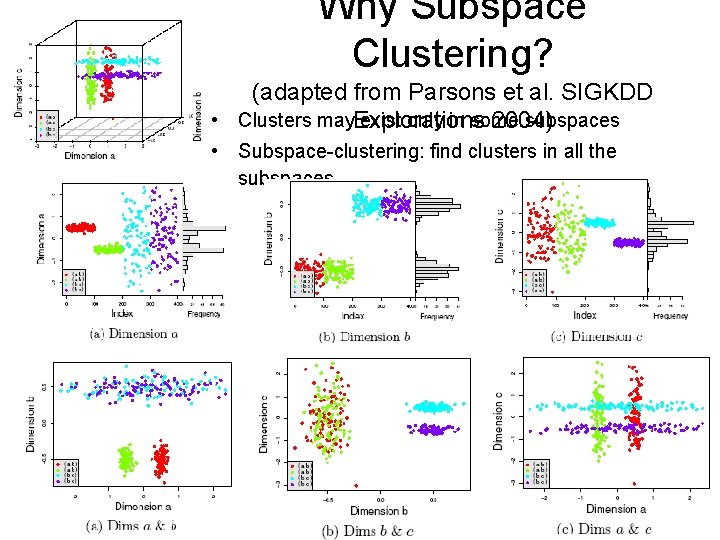Why Subspace Clustering? (adapted from Parsons et al. SIGKDD • Clusters may exist only in some subspaces Explorations 2004) • Subspace-clustering: find clusters in all the subspaces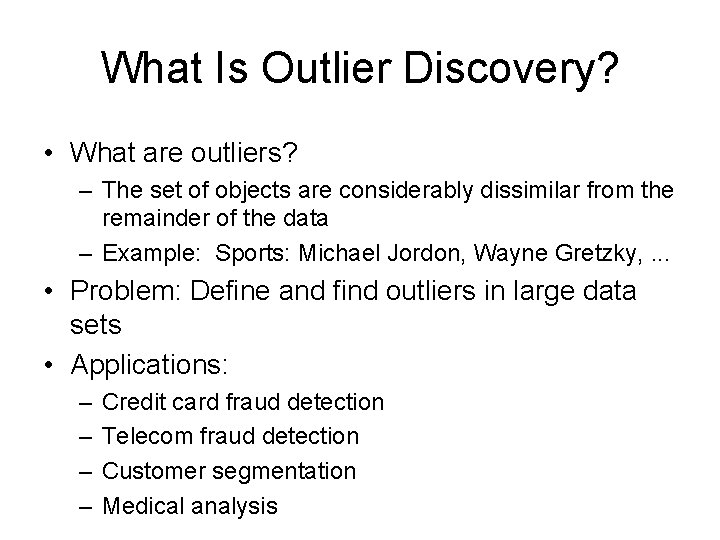What Is Outlier Discovery? • What are outliers? – The set of objects are considerably dissimilar from the remainder of the data – Example: Sports: Michael Jordon, Wayne Gretzky, . . . • Problem: Define and find outliers in large data sets • Applications: – – Credit card fraud detection Telecom fraud detection Customer segmentation Medical analysisOutlier Discovery: Statistical Approaches f. Assume a model underlying distribution that generates data set (e. g. normal distribution) • Use discordancy tests depending on – data distribution – distribution parameter (e. g. , mean, variance) – number of expected outliers • Drawbacks – most tests are for single attribute – In many cases, data distribution may not be known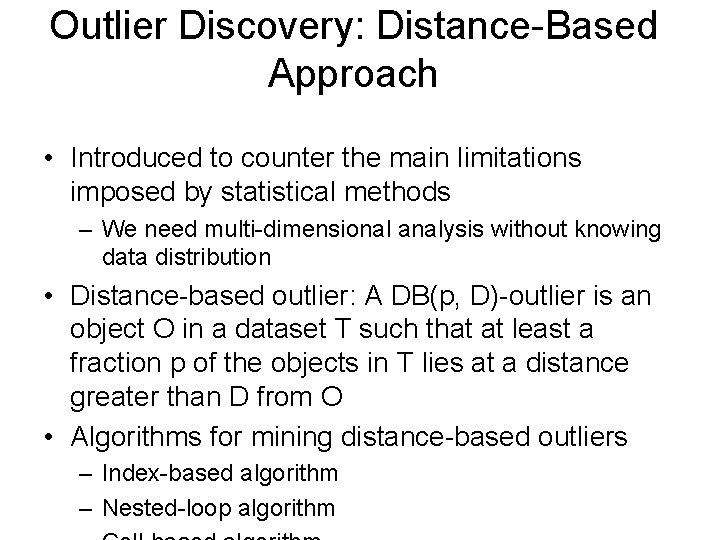Outlier Discovery: Distance-Based Approach • Introduced to counter the main limitations imposed by statistical methods – We need multi-dimensional analysis without knowing data distribution • Distance-based outlier: A DB(p, D)-outlier is an object O in a dataset T such that at least a fraction p of the objects in T lies at a distance greater than D from O • Algorithms for mining distance-based outliers – Index-based algorithm – Nested-loop algorithmDensity-Based Local Outlier Detection • Distance-based outlier detection is based on global distance distribution • It encounters difficulties to • Local outlier factor identify outliers if data is (LOF) not uniformly distributed – Assume outlier is not crisp • Ex. C 1 contains 400 loosely – Each point has a LOF distributed points, C 2 has 100 tightly condensed points, 2 outlier points o 1, o 2 • Distance-based method cannot identify o as an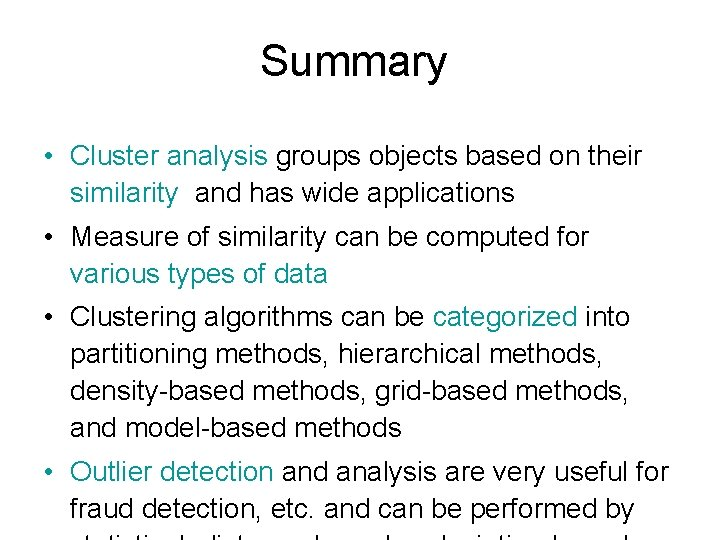Summary • Cluster analysis groups objects based on their similarity and has wide applications • Measure of similarity can be computed for various types of data • Clustering algorithms can be categorized into partitioning methods, hierarchical methods, density-based methods, grid-based methods, and model-based methods • Outlier detection and analysis are very useful for fraud detection, etc. and can be performed byReferences (1) • R. Agrawal, J. Gehrke, D. Gunopulos, and P. Raghavan. Automatic subspace clustering of high dimensional data for data mining applications. SIGMOD'98 • M. R. Anderberg. Cluster Analysis for Applications. Academic Press, 1973. • M. Ankerst, M. Breunig, H. -P. Kriegel, and J. Sander. Optics: Ordering points to identify the clustering structure, SIGMOD’ 99. • P. Arabie, L. J. Hubert, and G. De Soete. Clustering and Classification. World Scientific, 1996 • Beil F. , Ester M. , Xu X. : "Frequent Term-Based Text Clustering", KDD'02 • M. M. Breunig, H. -P. Kriegel, R. Ng, J. Sander. LOF: Identifying Density-Based Local Outliers. SIGMOD 2000. • M. Ester, H. -P. Kriegel, J. Sander, and X. Xu. A density-based algorithm for discovering clusters in large spatial databases. KDD'96. • M. Ester, H. -P. Kriegel, and X. Xu. Knowledge discovery in large spatial databases: Focusing techniques for efficient class identification. SSD'95. • D. Fisher. Knowledge acquisition via incremental conceptual clustering. Machine Learning, 2: 139 -172, 1987.References (2) • V. Ganti, J. Gehrke, R. Ramakrishan. CACTUS Clustering Categorical Data Using Summaries. KDD'99. • D. Gibson, J. Kleinberg, and P. Raghavan. Clustering categorical data: An approach based on dynamic systems. In Proc. VLDB’ 98. • S. Guha, R. Rastogi, and K. Shim. Cure: An efficient clustering algorithm for large databases. SIGMOD'98. • S. Guha, R. Rastogi, and K. Shim. ROCK: A robust clustering algorithm for categorical attributes. In ICDE'99, pp. 512 -521, Sydney, Australia, March 1999. • A. Hinneburg, D. l A. Keim: An Efficient Approach to Clustering in Large Multimedia Databases with Noise. KDD’ 98. • • A. K. Jain and R. C. Dubes. Algorithms for Clustering Data. Printice Hall, 1988. G. Karypis, E. -H. Han, and V. Kumar. CHAMELEON: A Hierarchical Clustering Algorithm Using Dynamic Modeling. COMPUTER, 32(8): 68 -75, 1999. L. Kaufman and P. J. Rousseeuw. Finding Groups in Data: an Introduction to Cluster Analysis. John Wiley & Sons, 1990. • • E. Knorr and R. Ng. Algorithms for mining distance-based outliers in large datasets. VLDB’ 98. • G. J. Mc. Lachlan and K. E. Bkasford. Mixture Models: Inference and Applications to Clustering. John Wiley and Sons, 1988.References (3) • L. Parsons, E. Haque and H. Liu, Subspace Clustering for High Dimensional Data: A Review , SIGKDD Explorations, 6(1), June 2004 • E. Schikuta. Grid clustering: An efficient hierarchical clustering method for very large data sets. Proc. 1996 Int. Conf. on Pattern Recognition, . • G. Sheikholeslami, S. Chatterjee, and A. Zhang. Wave. Cluster: A multi-resolution clustering approach for very large spatial databases. VLDB’ 98. • A. K. H. Tung, J. Han, L. V. S. Lakshmanan, and R. T. Ng. Constraint-Based Clustering in Large Databases, ICDT'01. • A. K. H. Tung, J. Hou, and J. Han. Spatial Clustering in the Presence of Obstacles , ICDE'01 • H. Wang, W. Wang, J. Yang, and P. S. Yu. Clustering by pattern similarity in large data sets, SIGMOD’ 02. • W. Wang, Yang, R. Muntz, STING: A Statistical Information grid Approach to Spatial Data Mining, VLDB’ 97. • T. Zhang, R. Ramakrishnan, and M. Livny. BIRCH : an efficient data clustering method for very large databases. SIGMOD'96.